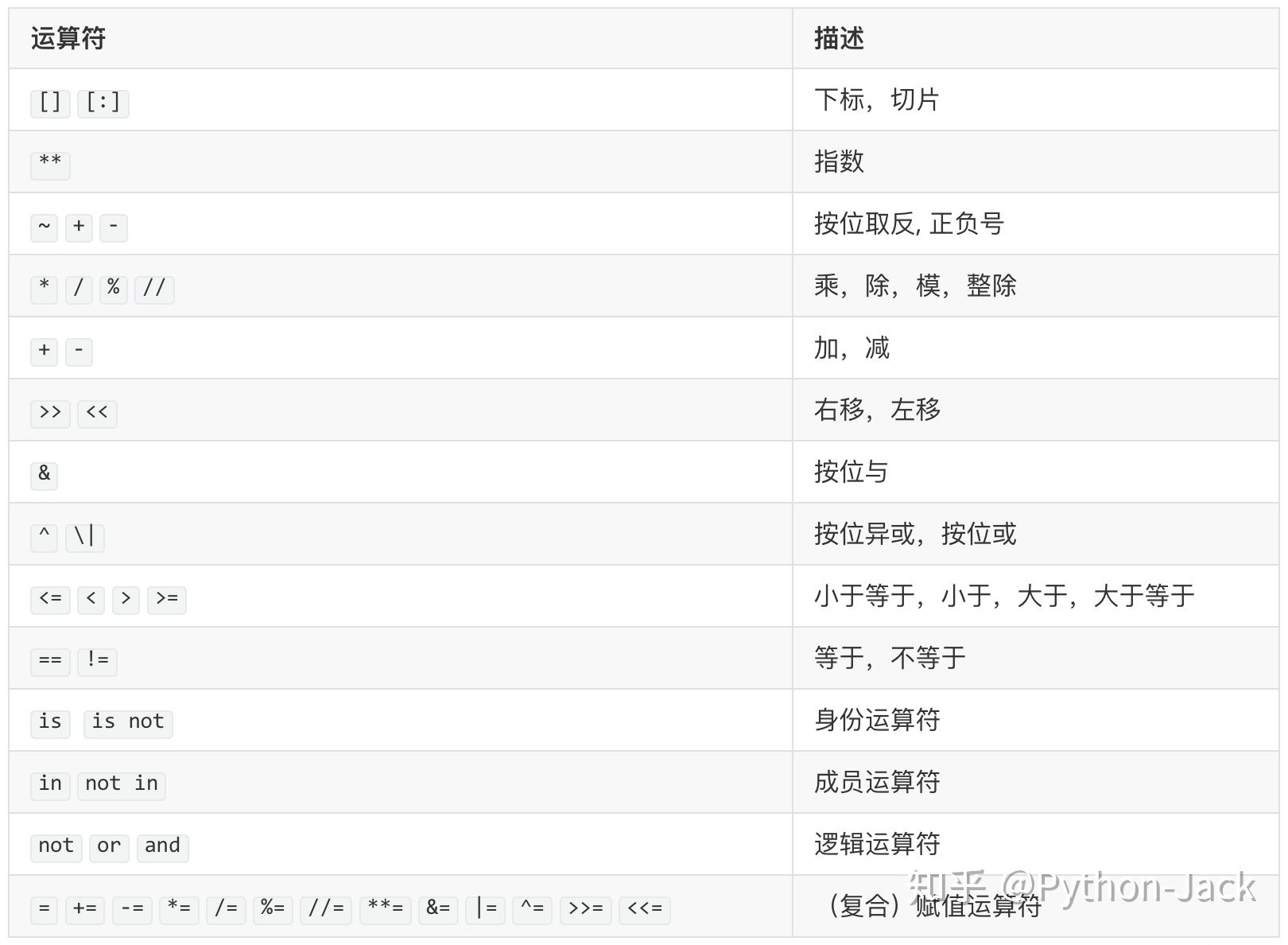# 从零开始学Python系列-第4讲：Python语言元素之运算符

Python支持多种运算符，我们先用一个表格为大家列出几乎所有的运算符，然后再选择一些比较重要的运算符为大家进行讲解。### 算术运算符

Python中的算术运算符非常丰富，除了大家最为熟悉的加减乘除之外，还有整除运算符、求模（求余数）运算符和求幂运算符。下面的例子为大家展示了算术运算符的使用。

"""

Version: 0.1
Author: 骆昊
"""
print(321 + 123)     # 加法运算
print(321 - 123)     # 减法运算
print(321 * 123)     # 乘法运算
print(321 / 123)     # 除法运算
print(321 % 123)     # 求模运算
print(321 // 123)    # 整除运算
print(321 ** 123)    # 求幂运算

### 赋值运算符

"""

Version: 0.1
Author: 骆昊
"""
a = 10
b = 3
a += b        # 相当于：a = a + b
a *= a + 2    # 相当于：a = a * (a + 2)
print(a)      # 算一下这里会输出什么

### 比较运算符和逻辑运算符

"""

Version: 0.1
Author: 骆昊
"""
flag0 = 1 == 1
flag1 = 3 > 2
flag2 = 2 < 1
flag3 = flag1 and flag2
flag4 = flag1 or flag2
flag5 = not (1 != 2)
print('flag0 =', flag0)    # flag0 = True
print('flag1 =', flag1)    # flag1 = True
print('flag2 =', flag2)    # flag2 = False
print('flag3 =', flag3)    # flag3 = False
print('flag4 =', flag4)    # flag4 = True
print('flag5 =', flag5)    # flag5 = False

### 例子1：华氏温度转换为摄氏温度。

"""

Version: 0.1
Author: 骆昊
"""
f = float(input('请输入华氏温度: '))
c = (f - 32) / 1.8
print('%.1f华氏度 = %.1f摄氏度' % (f, c))

print(f'{f:.1f}华氏度 = {c:.1f}摄氏度')

### 例子2：输入圆的半径计算计算周长和面积。

"""

Version: 0.1
Author: 骆昊
"""
import math

perimeter = 2 * math.pi * radius
print('周长: %.2f' % perimeter)
print('面积: %.2f' % area)

### 例子3：输入年份判断是不是闰年。

"""

Version: 0.1
Author: 骆昊
"""
year = int(input('请输入年份: '))
is_leap = year % 4 == 0 and year % 100 != 0 or year % 400 == 0
print(is_leap)

### 总结